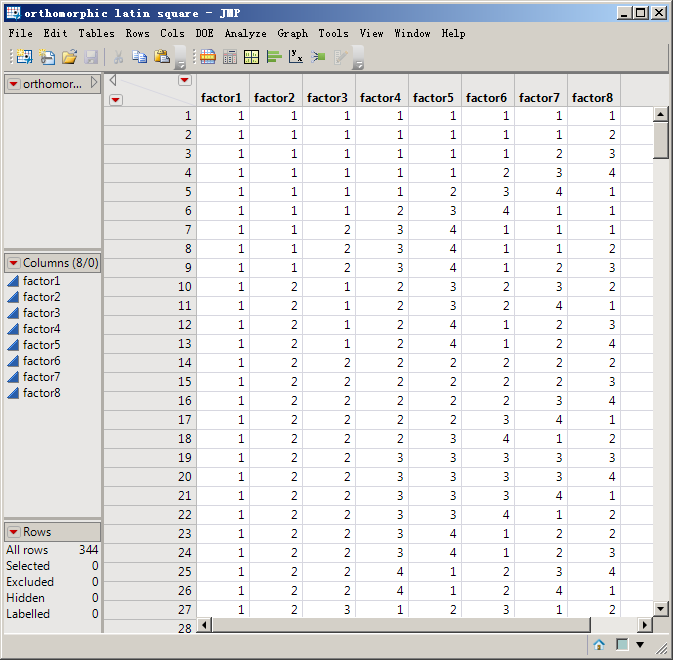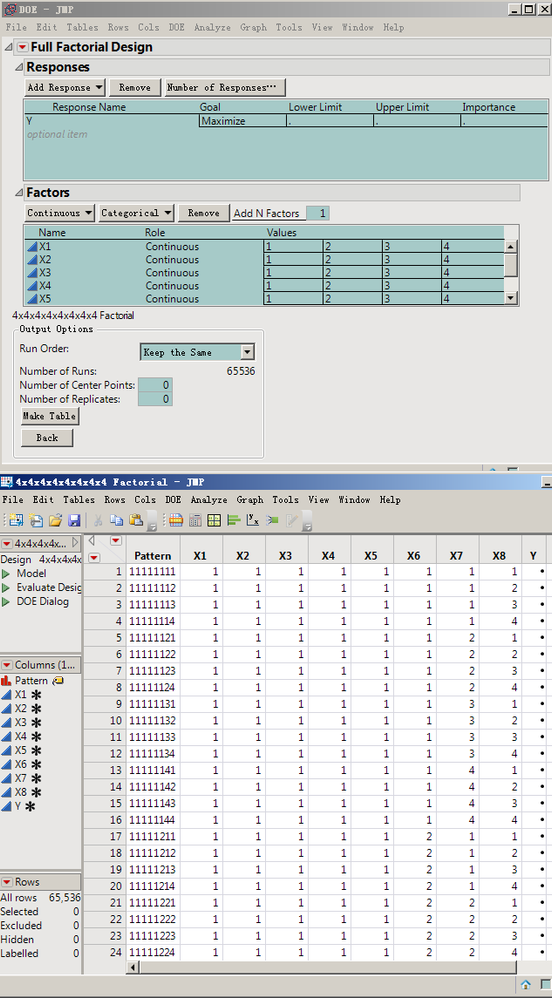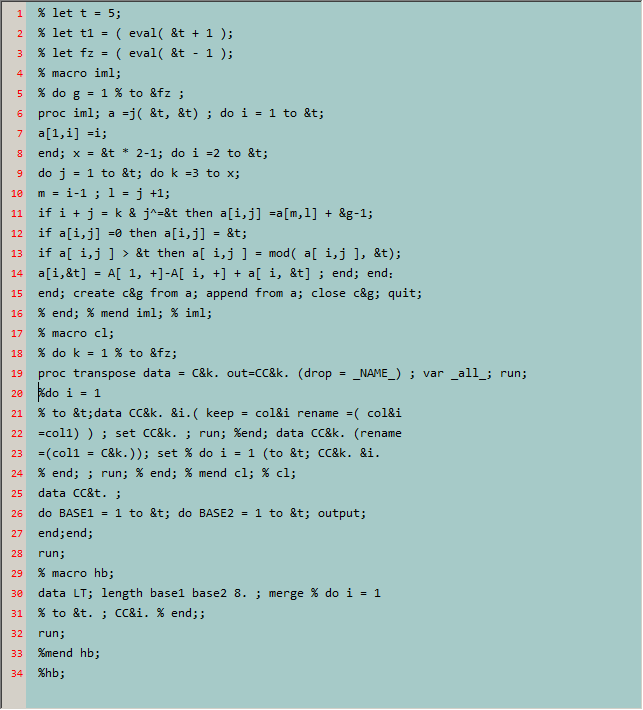Choose Language Hide Translation Bar
Highlighted

## How to use JSL to design orthomorphic latin square tables?

A complete design is required and the form obtained with DOE is incomplete.
For example, this is an 8-factor, 4-level design table.
Thanks!5 REPLIES 5
Highlighted

## Re: How to use JSL to design orthomorphic latin square tables?

Do you mean a full factorial design, 8 factors each 4 Level? This means 4^8 Settings = 65536,

JMP does exactly this, see the script. BR

``````DOE(
Full Factorial Design,
{Add Response( Maximize, "Y", ., ., . ),
Add Factor( Continuous, {1, 2, 3, 4}, "X1", 0 ),
Add Factor( Continuous, {1, 2, 3, 4}, "X2", 0 ),
Add Factor( Continuous, {1, 2, 3, 4}, "X3", 0 ),
Add Factor( Continuous, {1, 2, 3, 4}, "X4", 0 ),
Add Factor( Continuous, {1, 2, 3, 4}, "X5", 0 ),
Add Factor( Continuous, {1, 2, 3, 4}, "X6", 0 ),
Add Factor( Continuous, {1, 2, 3, 4}, "X7", 0 ),
Add Factor( Continuous, {1, 2, 3, 4}, "X8", 0 ),
Make Design, Simulate Responses( 0 ), Set Run Order( Keep the Same ), Make Table
}
)``````

Georg
Highlighted

## Re: How to use JSL to design orthomorphic latin square tables?

The result of this method is an exhaustive list of combinations.

4^8 = 65536Highlighted

## Re: How to use JSL to design orthomorphic latin square tables?

This is much more than the number of conformal Latin squares.

Highlighted

## Re: How to use JSL to design orthomorphic latin square tables?

JMP does not directly create these designs using any of the DOE platforms. See this reference.

On the other hand, JMP produces very many useful designs. Perhaps another design would satisfy your need. Why do you require the orthomorphic Latin square design? Why will another design not satisfy your need?

Learn it once, use it forever!
Highlighted

## Re: How to use JSL to design orthomorphic latin square tables?

I rewrote the sas code as VBA.
Can generate common orthogonal tables.But when  want to generate an orthogonal table of any factor, at any level,  Get a repetition when it's not prime.

Article Labels

There are no labels assigned to this post.